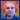Learn
Why Classic?
Start here to get the lowdown on Etherum Classic's reason for being and unique value proposition
Knowledge
Further reading on the foundations that underpin ETC
Guides
Practical tutorials and walkthroughs for interacting with the network in a number of ways
Videos
A collection of videos and podcasts to keep you informed on ETC concepts and happenings
Ethereum Classic Blog

# A Simple Interpretation Of The Ethereum Classic Mining Difficulty Parameter

Christian SeberinoThe Ethereum Classic (ETC) mining difficulty parameter may seem mysterious to some. I will show it has a beautiful easy to understand interpretation.

### .css-1r8a28j{-webkit-text-decoration:none!important;text-decoration:none!important;}.css-1r8a28j:hover{-webkit-text-decoration:underline!important;text-decoration:underline!important;}Background

Some ETC network computers receive rewards for creating, distributing and validating the blocks of the ETC blockchain. This work is referred to as mining and the computers as miners. To create valid blocks, miners keep adjusting random numbers in those blocks until corresponding 256 bit hashes meet a certain requirement. The random numbers are referred to as nonces. The hash requirements involve a number referred to as the difficulty. For a hash value H and difficulty d, this is the requirement:

H ≤ 2²⁵⁶ / d

### Interpretation

The probability of an arbitrary hash meeting the requirement is equal to the number of acceptable hashes divided by the number of possible hashes. In other words, for a difficulty d, this probability is equal to the following:

(2²⁵⁶ / d) / 2²⁵⁶ = 1 / d

I will now show that d equals the average required number of hashes to find an acceptable nonce. I will do this first using software and second using calculus:

Software Based Derivation - The following Python program finds the average required counts of random numbers, between zero and one, to obtain values less than 1 / d for various values of d:

``````#!/usr/bin/env python3

import random

ONE_MILLION = 1e6

def get_count(maximum):
"""
Calculates the number of random values, between 0 and 1,
generated before arriving at a value less than or equal to
some maximum.
"""

value = random.random()
count = 1
while value > maximum:
value  = random.random()
count += 1

return count

def get_average(maximum):
"""
Calculates the average output of one million get_count
invocations for a given maximum.
"""

average = 0
for i in range(int(ONE_MILLION)):
average += get_count(maximum) / ONE_MILLION

return average

print("difficulty        average")
print("=========================")
for d in [10, 500, 1000, 50000, 100000]:
average = get_average(1 / d)
print("   {:7d}     {:10.2f}".format(d, average))
``````

Here is the output:

``````difficulty        average
=========================
10          10.01
500         500.26
1000        1000.29
50000       49916.62
100000       99963.98
``````

The errors can always be made smaller by averaging over a larger quantity of numbers. Clearly the average count is the difficulty.

Calculus Based Derivation - The probablity of a hash count of one is 1 / d. The probability of a hash count of two is (1 / d)(1 - 1 / d). The probability of a hash count of three is (1 / d)(1 - 1 / d)², etc. The average hash count is the sum of the products of these probabilities and their corresponding hash counts:

(1 / d)(1) + (1 / d)(1 - 1 / d)(2) + (1 / d)(1 - 1 / d)² (3) + …

Let A(x) be a function based on this expression such that the average hash count is A(1):

A(x) = (1 / d) + (2 / d)(1 - 1 / d)x + (3 / d)(1 - 1 / dx² + …

Here is the antiderivative of A(x):

(1 / d)x + (1 / d)(1 - 1 / d)x² + (1 / d)(1 - 1 / dx³ + …

This is equal the following geometric series divided by d - 1:

(1 - 1 / d) x + (1 - 1 / dx² +(1 - 1 / dx³ + (1 - 1 / d)⁴ x⁴ + …

Using the formula for an infinite geometric series, this can be shown to equal:

d / (d + x - dx) - 1

Since A(x) is equal to the derivative of its antiderivative, A(x) is equal to the derivative of the above expression divided by d - 1:

A(x) = d / (d + x - dx

Clearly the average hash count A(1) = d.

### Conclusion

The mining difficulty can be understood as the average number of hashes required to find a valid nonce. If it was mysterious before, hopefully now it is less so.

### Feedback

You can contact me by clicking any of these icons:

### Acknowledgements

I would like to thank IOHK (Input Output Hong Kong) for funding this effort.cseberino
The ETC community is active on Discord
Github
Discord
Reddit
Made with `<3` for the Original Ethereum Vision